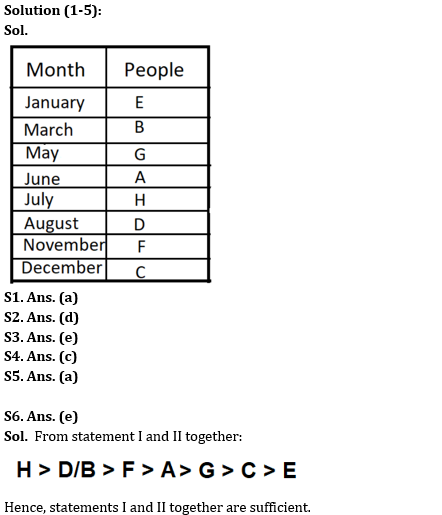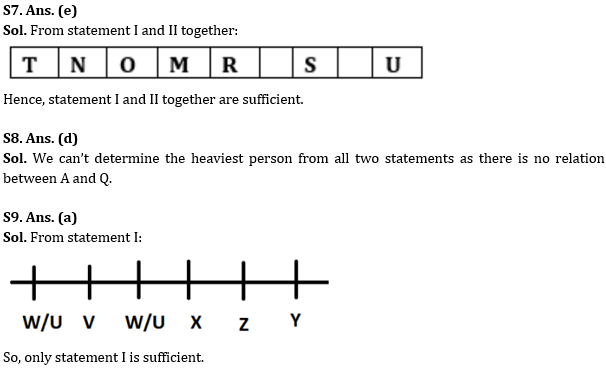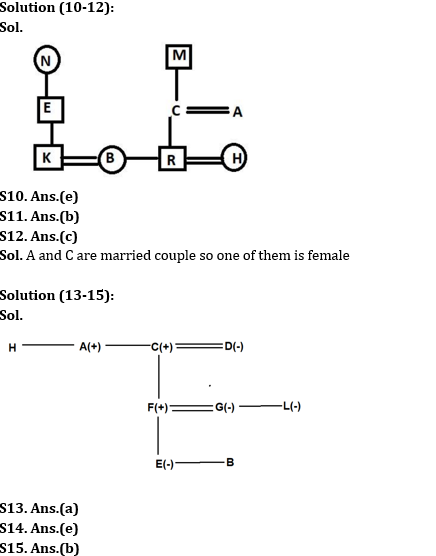Latest Banking jobs   »

# Reasoning Ability Quiz For RBI Grade B/ ECGC PO/ SIDBI Grade A Prelims 2022- 20ty May

Directions (1-5): Study the following information carefully and answer the given questions.
Eight people i.e. A, B, C, D, E, F, G, and H born in eight different months of the same year. These months are January, March, May, June, July, August, November, and December. Two persons born between G and the one who born in August. Not more than one person born between G and E. Three people born between E and H. A born just before H but does not born after July. More than two persons born between C and A. The one, who born in August, is not F and B. More than one person born between B and H. B born before F.

Q1. In which of the following month D born?
(a) August
(b) November
(c) June
(d) July
(e) None of these

Q2. Who among the following born in March?
(a) E
(b) A
(c) D
(d) B
(e) H

Q3. How many people born between B and C?
(a) Six
(b) Four
(c) Two
(d) Three
(e) Five

Q4. Which of the following statement is false?
(a) A born just after G
(b) Two people born between B and H
(c) F born in December
(d) H born in July
(e) None is false

Q5. Four of the following five alike in a certain way and hence form a group. Which one does not belong to the group?
(a) F
(b) D
(c) H
(d) C
(e) B

Directions (6-9): The following question is followed by two statements numbered I, and II. You have to decide, if these statements are sufficient to answer the asked questions and mark the correct option.

Q6. Eight persons A, B, C, D, E, F, G, and H are having different ages. Who among the following is the eldest?
Statements:
I. F is elder to A but younger to B and D. Neither B nor D is the eldest. G is elder to C.
II. C is only elder to E. B is elder to F. H is elder to A. A is elder to G.

(a) Only statement I is sufficient.
(b) Only statement II is sufficient.
(c) Either statement I or statement II is sufficient.
(d) Neither statement I nor statement II is sufficient.
(e) Both statements I and II together is sufficient.

Q7. Nine persons M, N, O, P, Q, R, S, T, and U are sitting in a straight line and facing north direction. Who among the following is sitting exactly in the middle?
Statements:
I. N is 2nd to the left of M. Two persons are sitting between M and S. U is 2nd to the right of S.
II. T is 2nd to the left of O. O is neighbor of M. R is 2nd to the left of S.

(a) Only statement I is sufficient.
(b) Only statement II is sufficient.
(c) Either statement I or statement II is sufficient.
(d) Neither statement I nor statement II is sufficient.
(e) Both statements I and II together is sufficient.

Q8. Who among P, Q, A, and B is the heaviest?
Statements:
I. Q is heavier than B.
II. P is not as heavy as either A or Q.

(a) Only statement I is sufficient.
(b) Only statement II is sufficient.
(c) Either statement I or statement II is sufficient.
(d) Neither statement I nor statement II is sufficient.
(e) Both statements I and II together are sufficient.

Q9. Six persons U, V, W, X, Y and Z are sitting in a row facing north. Who sits to the immediate right of X?
Statements:
I. Two persons sit between V and Z. Y sits to the immediate right of Z. V does not sit at any end. X sits adjacent to Z but not in the end.
II. U sits second to the left of Z. Only two persons sit to the left of U.

Directions (10-12): Read the following information carefully and answer the questions given below.
A three-generation family consists of nine members i.e., E, A, C, M, N, B, H, R and K. N is the mother of E. R is the child of C. H is the daughter-in-law of A. B is the sister-in-law of H. M is the father-in-law of A. E is the father-in-law of B. H is not the child of E. A has only one daughter.

Q10. What is the relation of C with respect to H?
(a) Father-in-law
(b) Mother-in-law
(c) Uncle
(d) Mother
(e) None of these

Q11. If A is the daughter-in-law of P then what is the relation of P with respect to B?
(a) Maternal grandmother
(b) Paternal grandmother
(c) Paternal aunt
(d) Maternal aunt
(e) None of these

Q12. How many female members are there in the family?
(a) Six
(b) Five
(c) Four
(d) Three
(e) None of these

Directions (13-15): Read the following information carefully and answer the questions given below.
A is the unmarried brother of C. C is the father-in-law of G who is the mother of two children. D is the sister-in-law of H. D has no siblings. F is the father of E who is the sister of B. L is the sister of G. A is the paternal uncle of F. D is the mother of F.

Q13. How is L related to E?
(a) Aunt
(b) Brother
(c) Brother-in-law
(d) Sister-in-law
(e) None of the above

Q14. How many female members are there in a family?
(a) 2
(b) 3
(c) 4
(d) 5
(e) Cannot be determined

Q15. Which of the following statement is false?
(a) L is the aunt of E
(b) C is the uncle of H
(c) L is the sister of G
(d) C is the father of F
(e) All of the above is false

Solutions#### Congratulations!